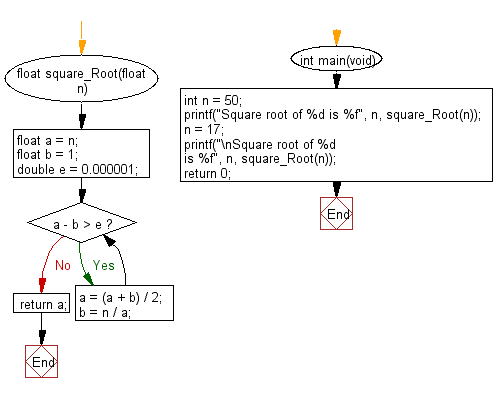﻿ C Program: Babylonian method to find square roots

# C Exercises: Find the square root of a number using Babylonian method

## C Programming Mathematics: Exercise-19 with Solution

Write a C program to find the square root of a number using the Babylonian method.

Example 1:
Input: n = 50
Output: 7.071068
Example 2:
Input: n = 17
Output: 4.123106

Sample Solution:

C Code:

``````#include <stdio.h>

float square_Root(float n)
{

float a = n;
float b = 1;
double e = 0.000001;
while (a - b > e) {
a = (a + b) / 2;
b = n / a;
}
return a;
}

int main(void)
{
int n = 50;
printf("Square root of %d is %f", n, square_Root(n));
n = 17;
printf("\nSquare root of %d is %f", n, square_Root(n));
return 0;
}
```
```

Sample Output:

```Square root of 50 is 7.071068
Square root of 17 is 4.123106
```

Flowchart:C Programming Code Editor:

Improve this sample solution and post your code through Disqus.

What is the difficulty level of this exercise?

Test your Programming skills with w3resource's quiz.

﻿